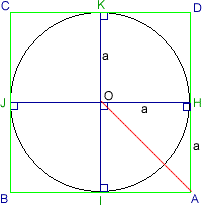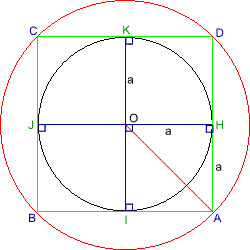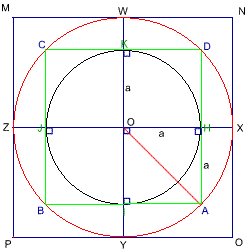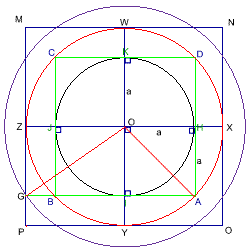#### You may also like### Some(?) of the Parts

A circle touches the lines OA, OB and AB where OA and OB are perpendicular. Show that the diameter of the circle is equal to the perimeter of the triangle### Triangle Incircle Iteration

Keep constructing triangles in the incircle of the previous triangle. What happens?### Medallions

Three circular medallions fit in a rectangular box. Can you find the radius of the largest one?

# Circle Scaling

##### Age 14 to 16 Challenge Level:

Many thanks to Junwei of BHASVIC school for the basis of this solution. A solution was also received from Andrei of School 205, Bucharest.First draw a circle, centre O and radius of a.

Draw two diameter lines inside the circle, and make sure these two lines are perpendicular.

From these two lines, we can draw four tangents to the circle which form a square ABCD touching the circle at HIJK.

Then OH = a = HA.Thus, OA equals $a\surd 2$.

Using the compasses with focus on O and length OA, make another circle which has an area two times the area of the original circle.Extend the original diameter lines to be the diameter lines for the new circle, and use the same method to form a new square MNOP which touches the new circle on WXYZ.

Thus,$OZ =a\surd 2.$Then extend AB to touch the bigger square's side PM on G. Since $OG^2 = OZ^2 + ZG^2 = 3^2$, then $OG = a \sqrt 3.$ Using the circle area formula, with radii of the three circles a, $a \sqrt 2$ and $a\sqrt 3$ respectively, we can easily work out that the ratio of their areas is 1:2:3.